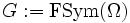Finitary symmetric group on countable set is subgroup-universal for finite groups

(diff) ← Older revision | Latest revision (diff) | Newer revision → (diff)
This article gives the statement, and possibly proof, of an embeddability theorem: a result that states that any group of a certain kind can be embedded in a group of a more restricted kind.
View a complete list of embeddability theorems

Statement

Let$\Omega$ be a countably infinite set and$G := \operatorname{FSym}(\Omega)$ be the finitary symmetric group on$\Omega$. Then,$G$ is subgroup-universal for finite groups. In other words, if$H$ is any finite group,$H$ is isomorphic to a subgroup of$G$.

Facts used

1. Cayley's theorem: This states that every group embeds as a subgroup of the symmetric group on its underlying set.

Proof

The proof follows from fact (1), and the observation that the symmetric group on any finite set is isomorphic to some subgroup of$G$ -- namely, the subgroup comprising the permutations on a finite subset of$\Omega$ of the same cardinality.